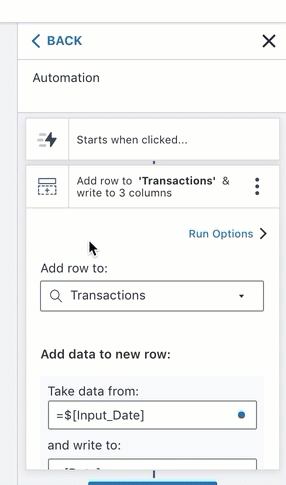# Inserting a cross-row formula in a table

Hi all, I'm having trouble with this "it's a bit spreadsheet, a bit database" table approach, and what you can and can't do with formulae.

For example if I have a classic Account Transactions table with columns for date (A), txn amount (B) and balance (C), in a spreadsheet the balance column would have a formula with relative references something like:

• for row 2: =C1 + B2
• for row 3: =C2 + B3
etc.

If I want to insert or copy a row from within an App Action, how could I write this formula correctly into the new row's balance column? I know that how I've described this simple example I could just write the calculated value in the balance column and not worry about the formula, but my real situation is a bit more complicated and needs this type of cross-row formula written into the cell.

Thanks

Hi @Stev-5755, thanks for the post and welcome to the community! Great question you have and I'm happy to share some ideas on what you can do here.

1. Build out a table like this. Note there are no formulas in any of the cells/columns. Rather, they are just formatted in date and currency respectively.

2. Below is an example app layout. Basically you have a few content boxes (Date, Amount, Total), two input field objects (Input_Date and Input_Amount), a button object (Submit), and then a data cell object. The input field objects are editable variables (I named them Input_Date and Input_Amount) and the total data cell has a formula of `=SUM(Transactions[Amount])`. This simply totals the Amount column in the Transactions.

3. The Submit button adds data to the three columns in the Transactions table4. And the final product is...

Here's the updated table

Like you mentioned, this is a simplified case but hopefully it provides some insight on how to design your table and app. If you have any more questions please don't hesitate to come back and visit us here!

Hi @aj, thanks for your response! That's a different way of looking at the problem, which is what I was after. As I alluded to though, my use case is a bit more complex and may preclude this approach. In reality Interest calculations are also involved which means the formula containing SUM(Transactions[Amount]) would also need to contain (\$[Input_Date] - previous-row's-date), i.e. still a cross-row reference, which I think maybe I can implement using FINDLASTROW() somehow.

But then there's a "wishlist" requirement I'd be interested in solving which is, to always have a last row after the latest transaction that has Date =TODAY() and Total =(an Interest calculation formula) so that the last row is a running total of Interest incurred at any time. This really would need a cross-row formula in the table.

Thanks!

Hi @Stev-5755,

Perhaps you could use a SUMIF formula to get the cumulative total:

=SUMIF([Date] < TODAY(), [Amount])

Thanks,
Matt

1 Like